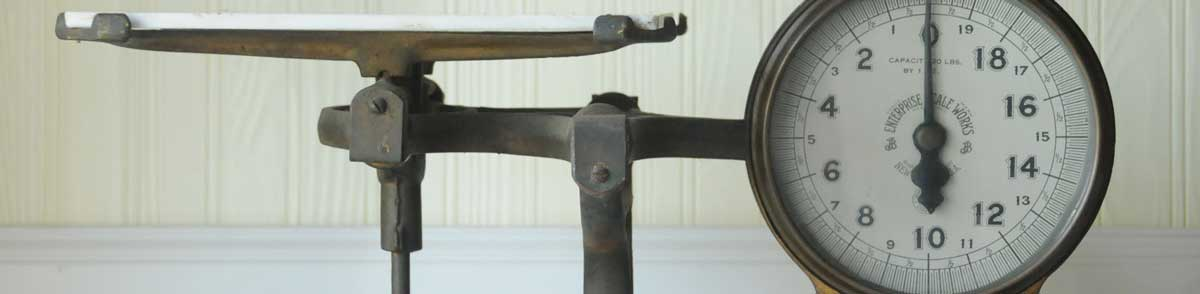# Pounds to Milligrams Conversion

Enter the weight in pounds below to get the value converted to milligrams.

lb
oz
Results in Milligrams:1 lb = 453,592.37 mg
453.59237 g

## How to Convert Pounds to MilligramsTo convert a pound measurement to a milligram measurement, multiply the weight by the conversion ratio.

Since one pound is equal to 453,592.37 milligrams, you can use this simple formula to convert:

milligrams = pounds × 453,592.37

The weight in milligrams is equal to the pounds multiplied by 453,592.37.

For example, here's how to convert 5 pounds to milligrams using the formula above.
5 lb = (5 × 453,592.37) = 2,267,961.85 mg

## Pounds

One pound is defined as a unit of mass/weight equal to 16 ounces, or 0.45359237 kilograms. One pound is equal to 7,000 grains in the avoirdupois or apothecaries' systems.

The pound is a US customary and imperial unit of weight. A pound is sometimes also referred to as a common ounce. Pounds can be abbreviated as lb, and are also sometimes abbreviated as lbs, lbm, or #. For example, 1 pound can be written as 1 lb, 1 lbs, 1 lbm, or 1 #.

## Milligrams

The milligram is the mass equal to 1/1,000 of a gram, or 0.0154 grains.

The milligram, or milligramme, is an SI unit of weight in the metric system. In the metric system, "milli" is the prefix for 10-3. Milligrams can be abbreviated as mg; for example, 1 milligram can be written as 1 mg.

## Pound to Milligram Conversion Table

Pound measurements converted to milligrams
Pounds Milligrams
0.00001 lb 4.5359 mg
0.00002 lb 9.0718 mg
0.00003 lb 13.61 mg
0.00004 lb 18.14 mg
0.00005 lb 22.68 mg
0.00006 lb 27.22 mg
0.00007 lb 31.75 mg
0.00008 lb 36.29 mg
0.00009 lb 40.82 mg
0.000001 lb 0.453592 mg
0.00001 lb 4.5359 mg
0.0001 lb 45.36 mg
0.001 lb 453.59 mg
0.01 lb 4,536 mg
0.1 lb 45,359 mg
1 lb 453,592 mg

## References

1. Encyclopædia Britannica, Avoirdupois weight, https://www.britannica.com/science/avoirdupois-weight